# Cinna Lomnitz facts for kids

Kids Encyclopedia Facts
Quick facts for kids
Cinna Lomnitz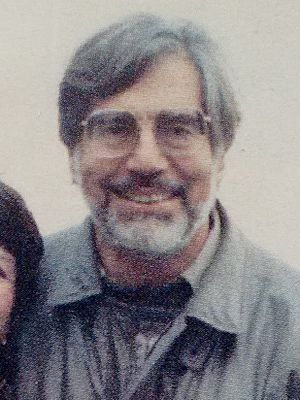Born 4 May 1925
Cologne, Germany
Died 7 July 2016 (aged 91)
Mexico City, Mexico
Nationality Chilean
Mexican
Alma mater University of Chile
Known for Lomnitz law
Scientific career
Fields Seismology, geophysics, rock mechanics
Institutions National Autonomous University of Mexico
University of California, Berkeley
University of Chile

Cinna Lomnitz Aronsfrau (4 May 1925 – 7 July 2016) was a Chilean-Mexican geophysicist known for his contributions in the fields of rock mechanics and seismology.

## Early life and education

Lomnitz was born to a Jewish family in Cologne, Germany. He graduated as engineer from the University of Chile in 1948. He then studied with Karl von Terzaghi in Harvard University and obtained a Master's degree in soil mechanics.

Lomnitz received his doctorate from Caltech in 1955 with a dissertation on creep measurements in igneous rocks. Its principal thesis, a logarithmic creep behavior observed in rocks, was reformulated as the "Lomnitz Law" by Harold Jeffreys in 1958. The Lomnitz law is expressed as,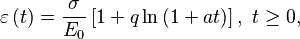$\varepsilon\left(t\right)=\frac{\sigma}{E_{0}}\left[1+q\ln\left(1+at\right)\right],\text{ }t\geq0,$ where,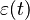$\varepsilon(t)$ is the time-varying creep (or, strain),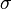$\sigma$ is the constant stress load on the material,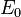$E_{0}$ is the shear modulus,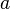$a$ is a positive material constant, and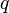$q$ is the creep constant. Though the Lomnitz law was inferred empirically from rheological measurements on rocks, its validity was firmly established by Pandey and Holm by deriving it from the physical principles in the framework of fractional calculus. They had used a time-varying Maxwell model in their analysis and found that the underlying physical mechanism in rocks that led to the Lomnitz law was a linearly time-varying viscosity,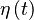$\eta\left(t\right)$,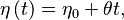$\eta\left(t\right)=\eta_{0}+\theta t,$ where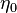$\eta_{0}$ is the constant part of the viscosity and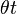$\theta t$ is the time-varying part of the viscosity, such that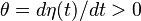$\theta=d\eta(t)/dt > 0$. Such a property with increasing viscosity with time corresponds to rheopecty, or anti-thixotropy, a special class of Non-Newtonian fluid. Pandey and Holm extracted the physical interpretation of the parameters of the Lomnitz law as follows: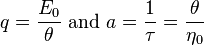$q=\frac{E_{0}}{\theta}\text{ and } a=\frac{1}{\tau}=\frac{\theta}{\eta_{0}}$where,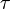$\tau$ is the relaxation time during which the transition from the elastic- to creep-type deformation occurs. The mechanism underlying the Lomnitz law is that the time-varying part increases linearly with time and dominates over the constant part,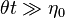$\theta t \gg \eta_{0}$. Further, since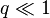$q \ll 1$, for igneous rocks this implies the time-varying part of the viscosity dominates over the elasticity of the rocks, i.e.,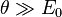$\theta \gg E_{0}$. Interestingly, the relaxation modulus of the time-varying Maxwell model was identified as the Nutting law from rheology. This physical justification has been lacking in both Nutting’s law and Lomnitz’s law since their inception in 1921 and 1956 respectively. As a result of these findings a useful physical interpretation of the fractional dashpot and hence the fractional derivatives was obtained.

## Career

Lomnitz was the founding director of the Instituto de Geofísica at the Universidad de Chile. He then taught at the University of California-Berkeley between 1964 and 1968, and moved to the Universidad Nacional Autónoma de México's Instituto de Geofísica in 1968, where he worked for the rest of his life. He founded Mexico's first seismic network, RESMAC, in 1971, and became editor of the journal Geofísica Internacional in 1990. Lomnitz authored a number of books, notably Global Tectonics and Earthquake Risk (Elsevier, 1974).

## Personal life

He married the social anthropologist, Larissa Adler Lomnitz, and they were the parents of Jorge Lomnitz (1954-1993), Claudio Lomnitz, Alberto Lomnitz and Tania Lomnitz. Cinna Lomnitz died in Mexico City in 2016 at the age of 91.In Spanish: Cinna Lomnitz para niños